# How to Calculate and Solve for the Area, Base and Height of a Triangle | The Calculator Encyclopedia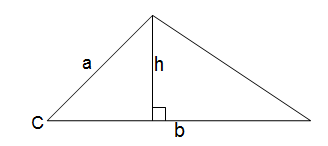The image above is a triangle.
To compute the area of a triangle two essential parameters are required which are the base of the triangle and the height of the triangle.

The formula for calculating the area of a triangle is:

A = 0.5bh

Where:

A = Area of a Triangle
b = Base of a Triangle
h = Height of a Triangle

Let’s solve an example:
Given that the base of a triangle is 12 cm and the height of the triangle is 4 cm. Find the area of the triangle.

This implies that:
b = Base of the Triangle = 12 cm
h = Height of the Triangle = 4 cm

A = 0.5bh
A = 0.5(12)(4)
A = 0.5(48)
A = 24

Therefore, the area of the triangle is 24 cm2

How to Calculate the Base of a Triangle when the Area and Height of the Triangle is Given

The formula is:

b = 2A / h

Where:

b = Base of the Triangle
A = Area of the Triangle
h = Height of the Triangle

Let’s take an example:
Find the base of a triangle when the height and area of the triangle is 12 cm and 40 cm2 respectively.

This implies that:
A = Area of the Triangle = 40 cm2
h = Height of the Triangle = 12 cm

b = 2(40) / 12
b = 80 / 12
b = 6.667

Therefore, the base of the triangle is 6.667 cm.

How to Calculate the Height of a Triangle when the Area and Base of the Triangle is Given

The formula is:

h = 2A / b

Where:

h = Height of the Triangle
A = Area of the Triangle
b = Base of the Triangle

Let’s take an example:
Find the height of a triangle when the base and area of the triangle is 20 cm and 80 cm2 respectively.

This implies that:
A = Area of the Triangle = 80 cm2
b = Base of the Triangle = 20 cm

h = 2(80) / 20
h = 160 / 20
h = 8

Therefore, the height of the triangle is 8 cm.

Nickzom Calculator – The Calculator Encyclopedia is capable of calculating the area of a triangle.

To get the answer and workings of the area of a triangle using the Nickzom Calculator – The Calculator Encyclopedia. First, you need to obtain the app.

You can get this app via any of these means:

To get access to the professional version via web, you need to register and subscribe for NGN 1,500 per annum to have utter access to all functionalities.
You can also try the demo version via https://www.nickzom.org/calculator

Once, you have obtained the calculator encyclopedia app, proceed to the Calculator Map, then click on Mensuration under the Mathematics section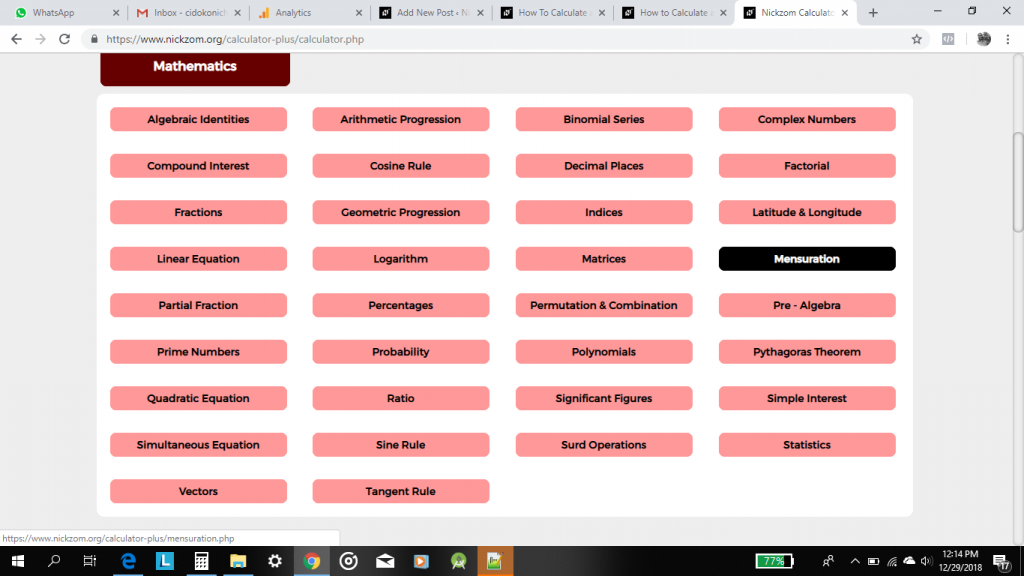Now, click on Area of a Triangle under Mensuration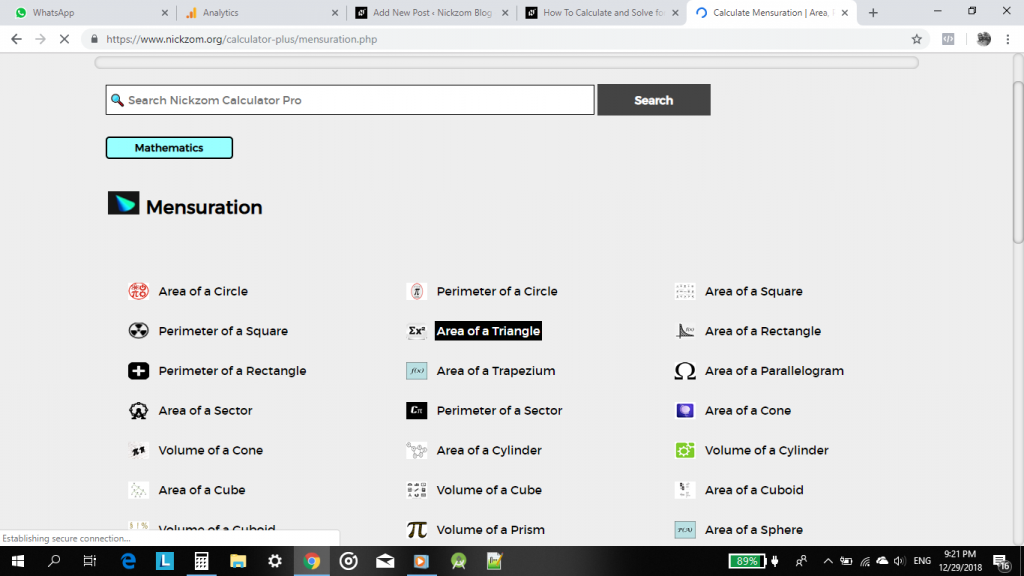Now, click on Area of a Triangle with No Angle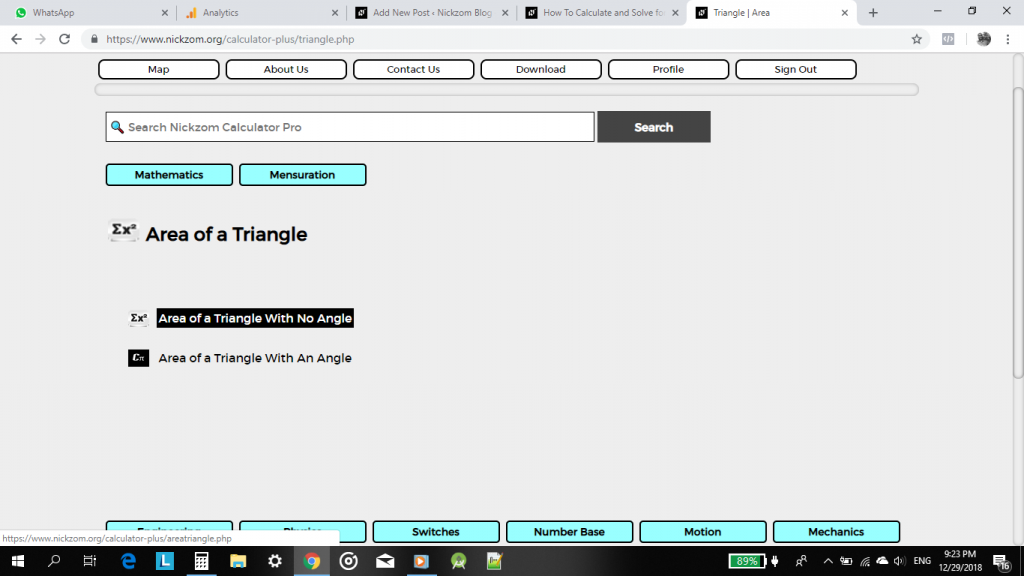The screenshot below displays the page or activity to enter your values, to get the answer for the area of a triangle according to the respective parameters which are the base of the triangle (b) and the height of the triangle (h).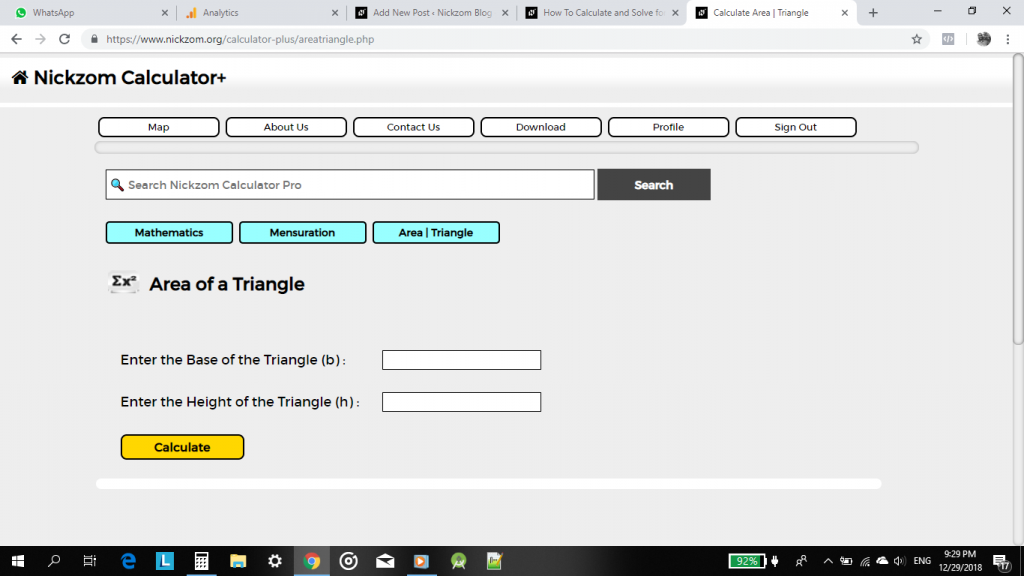Now, enter the values appropriately and accordingly for the parameters as required by the example above where the base of the triangle is 12 cm and the height of the triangle is 4 cm.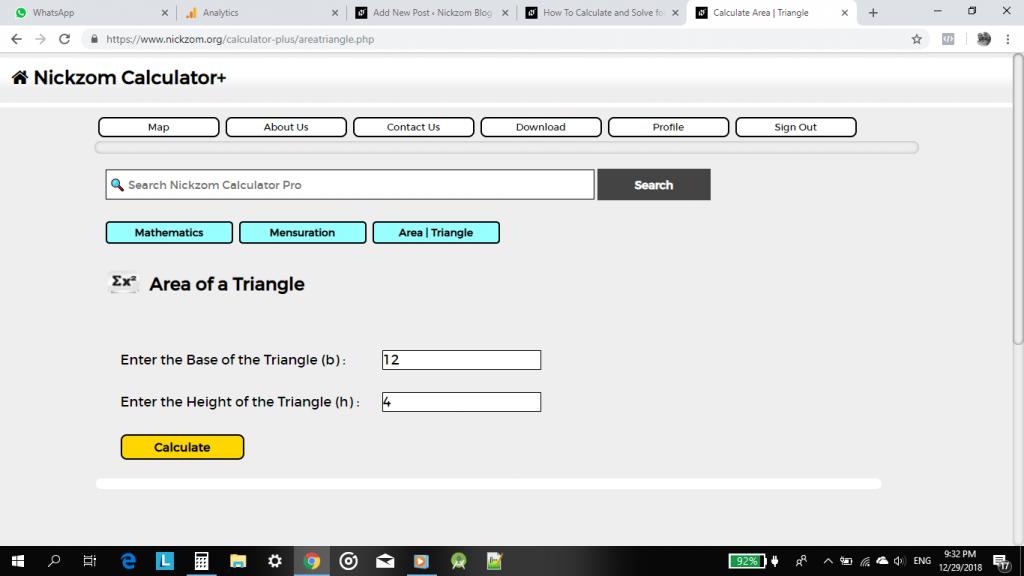Finally, click on Calculate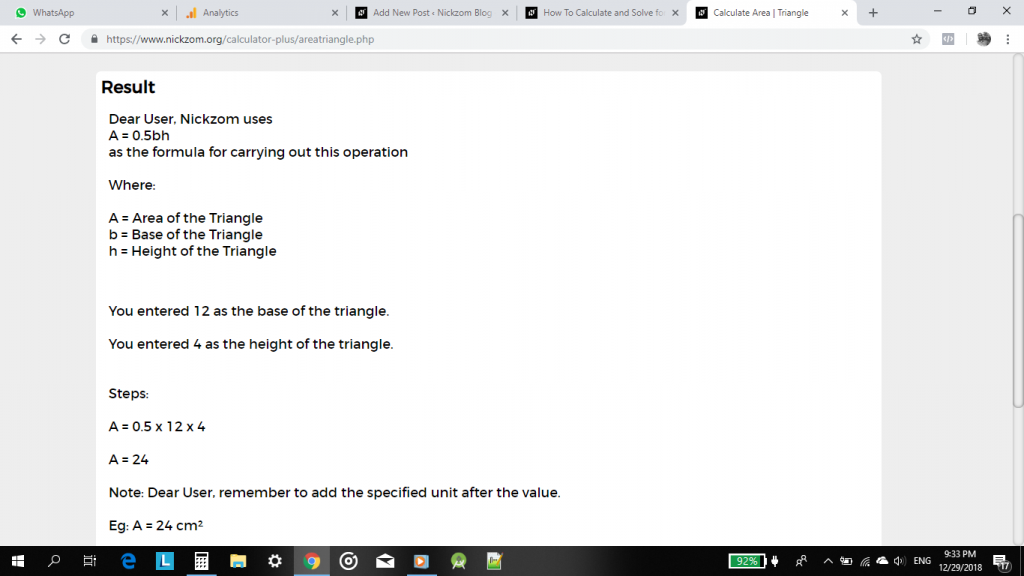As you can see from the screenshot above, Nickzom Calculator – The Calculator Encyclopedia solves for the area of a triangle and presents the formula, workings and steps too.